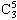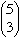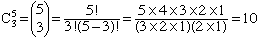Subject: Permutations and Combinations Name: Sarah Who is asking: Student Level: Secondary Question: Arnold has a dollar coin, one dime, one quarter, one nickel, and a penny. The number of different sums of money that can be formed using three coins is... Hi Sarah, Since each of the coins has a different value you get a different sum for each choice of three coins. Thus the number of different sums is the number of ways of selecting 3 coins from 5 coins. This quantity, called a binomial coefficient, is sometimes denotedand sometimesand its value isCan you write down all 10 different sums? Cheers, Penny Go to Math Central